# 图像分割与Watershed算法¶

### 代码¶import numpy as np
import cv2 as cv
from matplotlib import pyplot as plt
gray = cv.cvtColor(img,cv.COLOR_BGR2GRAY)
ret, thresh = cv.threshold(gray,0,255,cv.THRESH_BINARY_INV+cv.THRESH_OTSU)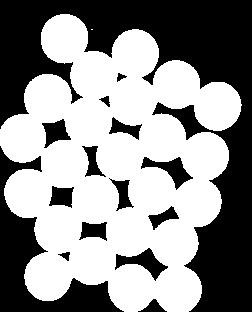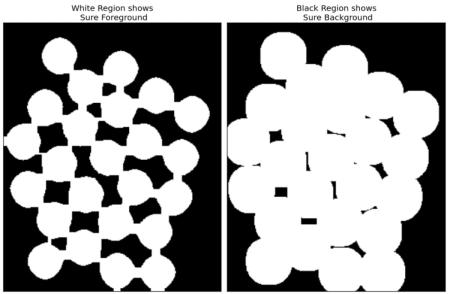# 噪声去除
kernel = np.ones((3,3),np.uint8)
opening = cv.morphologyEx(thresh,cv.MORPH_OPEN,kernel, iterations = 2)
# 确定背景区域
sure_bg = cv.dilate(opening,kernel,iterations=3)
# 寻找前景区域
dist_transform = cv.distanceTransform(opening,cv.DIST_L2,5)
ret, sure_fg = cv.threshold(dist_transform,0.7*dist_transform.max(),255,0)
# 找到未知区域
sure_fg = np.uint8(sure_fg)
unknown = cv.subtract(sure_bg,sure_fg)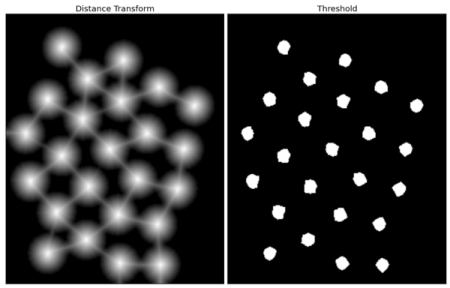# 类别标记
ret, markers = cv.connectedComponents(sure_fg)
# 为所有的标记加1，保证背景是0而不是1
markers = markers+1
# 现在让所有的未知区域为0
markers[unknown==255] = 0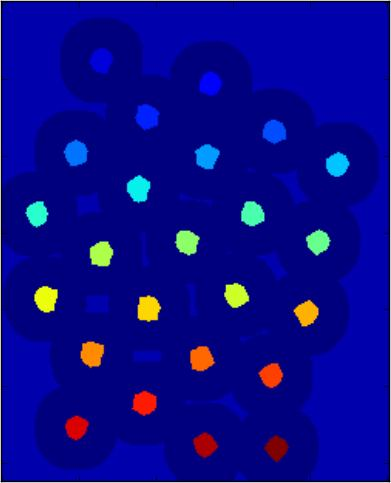markers = cv.watershed(img,markers)
img[markers == -1] = [255,0,0]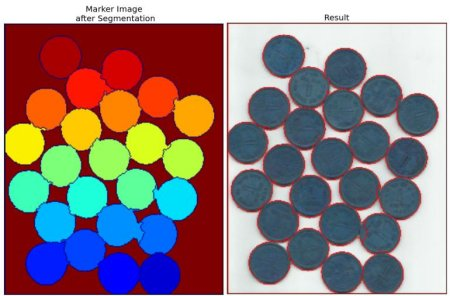### 附加资源¶

1. CMM page on Watershed Transformation

### 练习¶

1. OpenCV samples has an interactive sample on watershed segmentation, watershed.py. Run it, Enjoy it, then learn it.